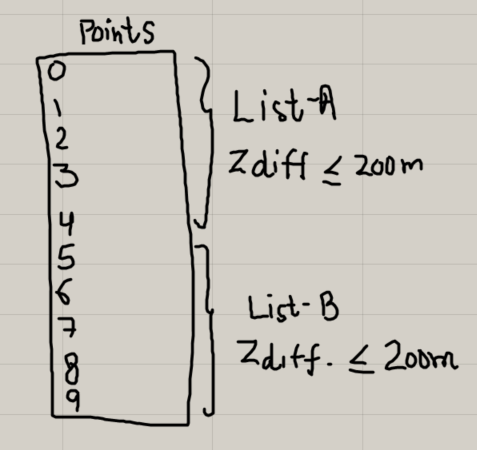# Divide list of sorted points into sub-lists based on Z-levels difference

Hi all,
I want to divide a list of sorted points into multiple list of points having maximum difference in "Z"levels say 200mm in that list such that they have the same sort order as in the native list.
Actually I want to use these points to create columns with uniform height keeping the maximum difference in Z-level limited to say 200mm only.
I have attached here a sample gh file and a picture for your referencePoints sorting_z levels_test.gh (375.0 KB)

Here is one idea, using python (I didn’t have some of your plugins, so reconnected your components a little differently, but I think it should still work for you):
Points sorting_z levels_test_v2.gh (389.2 KB)

``````import rhinoscriptsyntax as rs
import ghpythonlib.treehelpers as th

pnts = []
ind = []

def zrange(lst):
lst = [i.Z for i in lst]
ma = max(lst)
mi = min(lst)
dif = ma-mi
return dif

n = 0
while n < len(x):
pnts.append(x[n])
dif = zrange(pnts)
if dif>200:
n-=1
ind.append(n)
pnts = []
else:n+=1

def part(l, ind):
return [l[i:j] for i, j in zip(+ind, ind+[None])]

ol = part(x,ind)
a = th.list_to_tree(ol)
``````
1 Like

In line 19 , the index of the first item found that exceeds the desired maximum value is decremented.

Then in line 25, slice notation is used with the indices that were collected to divide the list of points into sublists. In python slice notation, the starting index is inclusive but the ending index is exclusive. For example, `list1[10:20]` will return a list including indices from 10 to 19 from `list1`

The combination of these two things has the effect that the index being decremented 2 times causing the last element to be skipped each time.

If you comment out or delete line 19 from your script, it will then have the desired result. I have commented it out here.

Points sorting_z levels_test_v2_re.gh (380.1 KB)

I also wrote a C# script to accomplish this.

Points sorting_z levels_test_221220a.gh (351.7 KB)

-Kevin

2 Likes

@kev.r Thank you for the response.
I tried to do it with Gh itself and compared the results with your script. Those are not matching somehow.At some point in the list there is change in the Z-level change order(Decreasing to increasing or vice versa).
Could you please have a look at it?
Points sorting_z levels_test_2212AY.gh (694.2 KB)

-Ayush

Hi,

I gave a try yesterday to this problem and ended up using the exact same method as you. The thing is, it’s not exactly the same thing as @kev.r script. What you are doing is filtering values by slices of size 200, whereas the C# script groups values until the domain exceeds 200. So your approach is less tolerant if you have big gaps in values. For instance, with the list below :
0
199
299
498

Your method would say : 0 and 199 are in the range (0 to 200(, so they go together. Then 299 in alone in the range (200,400(, then 498 is alone in (400,600(. Whereas Kevin’s approach would group 299 and 498 as they are less than 200 away, which I assume is what you want.

I really don’t think there is a solution without scripting, this is screaming for “while” loop that doesn’t exist in native Grasshopper.

1 Like

See attached as well

Clusters_PtsByZCoord_EntryLevel_V1.gh (116.9 KB)

1 Like